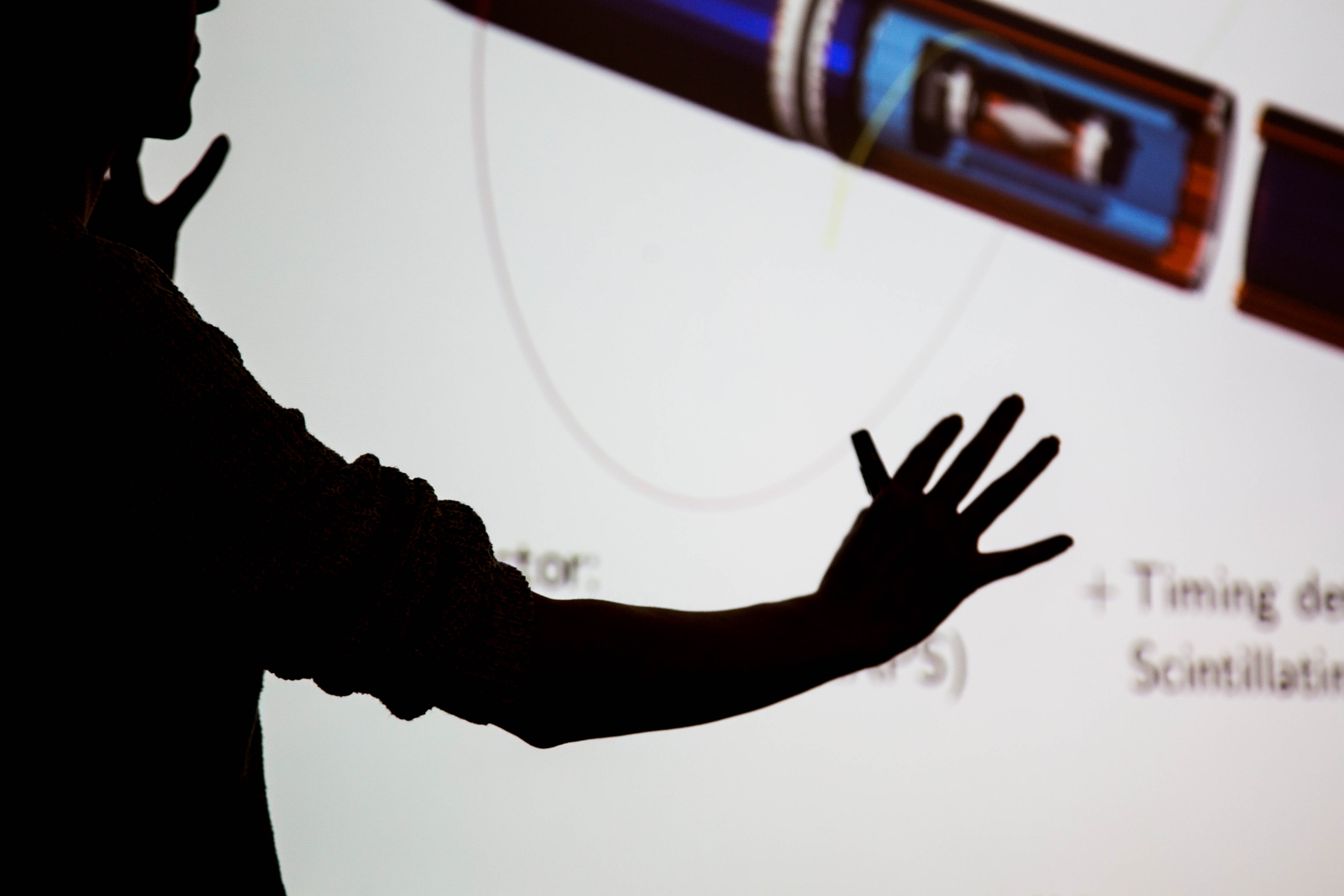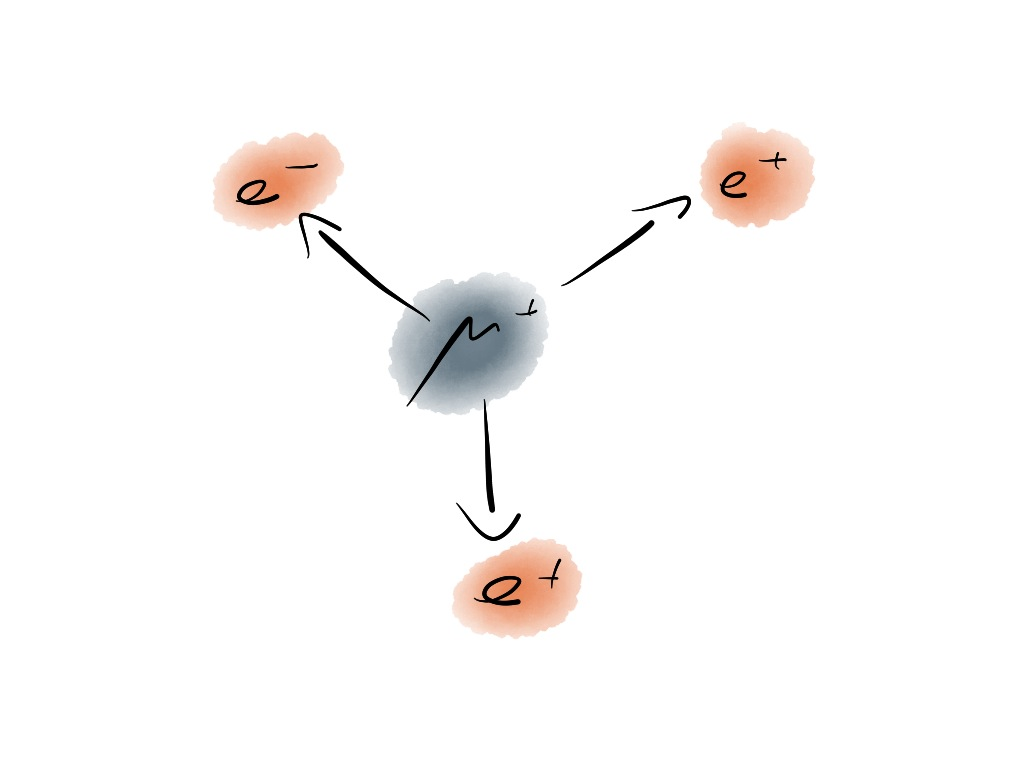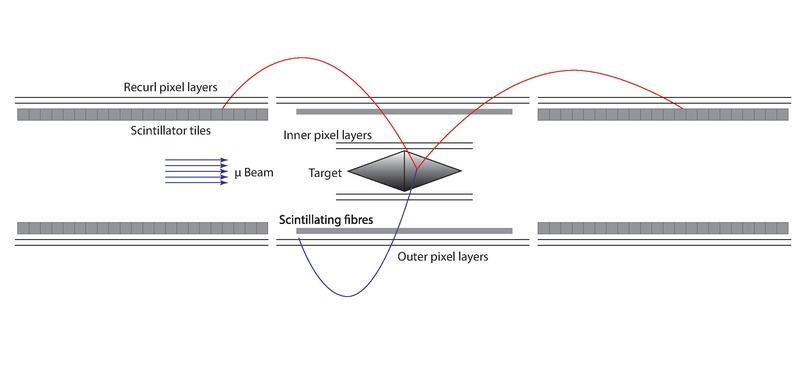## Mu3e Experiment

Searching for Lepton Flavour Violation
with utmost precision.

The observation of lepton flavour violation (LFV) would be a clear sign of physics beyond the Standard Model (SM). The Mu3e Collaboration aims to search for the LFV decay $$\mu\rightarrow eee$$ with a precision of one in $$10^{16}$$ muon decays.

### Mu3e Experiment

The Mu3e experiment is currently being built at the Paul-Scherrer Institut (PSI).#### Signal and Background

The invariant mass of $$\mu\rightarrow eee$$ signal decays equals the muon rest mass invariant mass $$m_{\mu}$$.
In background from rare SM muon decay $$\mu^+\rightarrow e^+e^-e^+\nu\nu$$, part of the energy is carried by the undetected neutrinos. Additional backgrounds stem from accidental combinations of three tracks.#### Detector

A high-intensity muon beam is stopped on a target. The tracks of the decay particles are measured in a lightweight tracking detector built from ultra-thin pixel sensors, scintillating fibres and tiles.

### Exotic muon decays @ ETP

At the ETP, we develop data acquisition for Mu3e and prepare the analysis for various exotic muon decays.